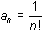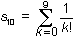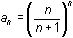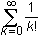Module 21 - Sequences and Series Introduction | Lesson 1 | Lesson 2 | Lesson 3 | Self-Test Self Test Use the seq( command to generate the first ten terms of the sequence, starting with n = 0. Find the exact value and a decimal approximation for the partial sum of the first 10 terms of the sequence given in Question 1:. Graph the first twenty terms of the sequencestarting with n = 1. Does the sequence appear to converge? If so, to what value does it converge? Make a table of values for the sequence. Use "Ask" mode to find a25, a50 and a100. Graph the sequence of partial sums forbeginning with the first partial sum and ending with the tenth partial sum. Does the sequence of partial sums appear to converge? If so, to what value does it converge? Graph the recursive sequence an = 0.75an-1 + 16; a0 = 16. Does the sequence appear to converge? If so, to what value does it converge? Click here to check your answers. < Back ©Copyright 2007 All rights reserved. | Trademarks | Privacy Policy | Link Policy